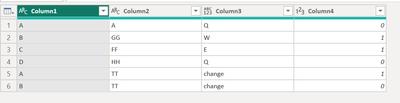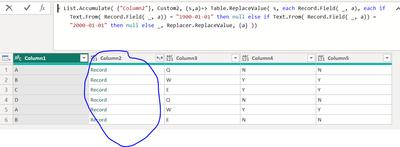cancel
Showing results for
Did you mean:Helper I

## Replace Numbers to Text in Power Query

Hi All, I have a sample table data where I am trying to replace 0 with N and 1 with Y using Replacevalue in power query. I cannot add as new custom column because, the same operation i should do on more than 40 columns. Table.``````let
Source = Table.FromRows(Json.Document(Binary.Decompress(Binary.FromText("i45WclTSAeNAIDZQitWJVnICstzdgUQ4EBuChZyBLDc3IOEKF3IBsjw8UDSCjAkJQdHoBBNyhaiKBQA=", BinaryEncoding.Base64), Compression.Deflate)), let _t = ((type nullable text) meta [Serialized.Text = true]) in type table [Column1 = _t, Column2 = _t, Column3 = _t, Column4 = _t]),
#"Changed Type" = Table.TransformColumnTypes(Source,{{"Column1", type text}, {"Column2", type text}, {"Column3", type text}, {"Column4", Int64.Type}}),
Custom1 = Table.ReplaceValue(#"Changed Type", each [Column3], each if ([Column1]="A" or [Column1]="B") and [Column2]="TT" then "change" else [Column3], Replacer.ReplaceValue, {"Column3"}),
Custom2 = Table.ReplaceValue(
Custom1,
each [Column4] = 0,
each "N",
(x,y,z)=> if y then z else x,
{"Column4"}
)
in
Custom2``````But i need to replace both 0 with N and 1 with Y in single power query step only.

Thanks,

Mohan V.

1 ACCEPTED SOLUTIONSuper User

Hi @Mohan128256 ,

Try this:

``````let
Source = Table.FromRows(Json.Document(Binary.Decompress(Binary.FromText("i45WclTSAeNAIDZQitWJVnICstzdgUQ4EBuChZyBLDc3IOEKF3IBsjw8UDSCjAkJQdHoBBNyhaiKBQA=", BinaryEncoding.Base64), Compression.Deflate)), let _t = ((type nullable text) meta [Serialized.Text = true]) in type table [Column1 = _t, Column2 = _t, Column3 = _t, Column4 = _t]),
#"Changed Type" = Table.TransformColumnTypes(Source,{{"Column1", type text}, {"Column2", type text}, {"Column3", type text}, {"Column4", Int64.Type}}),
Custom1 = Table.ReplaceValue(#"Changed Type", each [Column3], each if ([Column1]="A" or [Column1]="B") and [Column2]="TT" then "change" else [Column3], Replacer.ReplaceValue, {"Column3"}),

Custom2 =
Table.ReplaceValue(
Custom1,
each [Column4],
each if [Column4] = 0 then "N" else if [Column4] = 1 then "Y" else null,
Replacer.ReplaceValue,
{"Column4"}
)

in
Custom2``````

For this output:Pete

Now accepting Kudos! If my post helped you, why not give it a thumbs-up?

Proud to be a Datanaut!

6 REPLIES 6Solution Sage

Hi @Mohan128256 ,

You can give this a go.

``````let
Source = Table.FromRows(Json.Document(Binary.Decompress(Binary.FromText("i45WclTSAeNAIDZQitWJVnICstzdgUQ4EBuChZyBLDc3IOEKF3IBsjw8UDSCjAkJQdHoBBNyhaiKBQA=", BinaryEncoding.Base64), Compression.Deflate)), let _t = ((type nullable text) meta [Serialized.Text = true]) in type table [Column1 = _t, Column2 = _t, Column3 = _t, Column4 = _t]),
#"Changed Type" = Table.TransformColumnTypes(Source,{{"Column1", type text}, {"Column2", type text}, {"Column3", type text}, {"Column4", Int64.Type}}),
Custom1 = Table.ReplaceValue(#"Changed Type", each [Column3], each if ([Column1]="A" or [Column1]="B") and [Column2]="TT" then "change" else [Column3], Replacer.ReplaceValue, {"Column3"}),
#"Duplicated Column" = Table.DuplicateColumn(Custom1, "Column4", "Column5"),
Custom2 = List.Accumulate( {"Column4", "Column5"}, #"Duplicated Column", (s,a)=> Table.ReplaceValue( s, each Record.Field( _, a), each if Text.From( Record.Field( _, a)) = "0" then "N" else if Text.From( Record.Field( _, a)) = "1" then "Y" else _, Replacer.ReplaceValue, {a} ))
in
Custom2``````

You can provide it a list with column names and it will replace both 0 and 1's.Ps. If this helps solve your query please mark this post as Solution, thanks!Super User

This is actually a better answer, depending on your interpretation of "the same operation i should do on more than 40 columns".

If you interpret this as doing exactly the same thing to 40 columns, then this solution will allow you to list all the columns in one go, whereas my solution, as it is, won't.

Depending on the nature of the table data, this solution could be immensely powerful: you could potentially just feed it Table.ColumnNames and smash the whole table in one go, if every column fits the same data pattern. 👍

Pete

Now accepting Kudos! If my post helped you, why not give it a thumbs-up?

Proud to be a Datanaut!Helper I

@BA_Pete @m_dekorte how do we can manage the same for date data type columns?here 1900-01-01 and 2000-01-01 should be replaced as null else the dates.

I have tried this below but didnt work

``    Custom3 = List.Accumulate( {"Column2"}, Custom2, (s,a)=> Table.ReplaceValue( s, each Record.Field( _, a), each if Text.From( Record.Field( _, a)) = "1900-01-01" then null else if Text.From( Record.Field( _, a)) = "2000-01-01" then null else _, Replacer.ReplaceValue, {a} ))``Any help.Helper I

the code is bit complicate to understand.

But still thanks for the solution.Super User

Hi @Mohan128256 ,

Try this:

``````let
Source = Table.FromRows(Json.Document(Binary.Decompress(Binary.FromText("i45WclTSAeNAIDZQitWJVnICstzdgUQ4EBuChZyBLDc3IOEKF3IBsjw8UDSCjAkJQdHoBBNyhaiKBQA=", BinaryEncoding.Base64), Compression.Deflate)), let _t = ((type nullable text) meta [Serialized.Text = true]) in type table [Column1 = _t, Column2 = _t, Column3 = _t, Column4 = _t]),
#"Changed Type" = Table.TransformColumnTypes(Source,{{"Column1", type text}, {"Column2", type text}, {"Column3", type text}, {"Column4", Int64.Type}}),
Custom1 = Table.ReplaceValue(#"Changed Type", each [Column3], each if ([Column1]="A" or [Column1]="B") and [Column2]="TT" then "change" else [Column3], Replacer.ReplaceValue, {"Column3"}),

Custom2 =
Table.ReplaceValue(
Custom1,
each [Column4],
each if [Column4] = 0 then "N" else if [Column4] = 1 then "Y" else null,
Replacer.ReplaceValue,
{"Column4"}
)

in
Custom2``````

For this output:Pete

Now accepting Kudos! If my post helped you, why not give it a thumbs-up?

Proud to be a Datanaut!Helper I

Works like a charm.

Thanks,

Mohan V.Announcements#### Exclusive opportunity for Women!

Join us for a free, hands-on Microsoft workshop led by women trainers for women where you will learn how to build a Dashboard in a Day!#### Power Platform Conference-Power BI and Fabric Sessions

Join us Oct 1 - 6 in Las Vegas for the Microsoft Power Platform Conference.Top Solution Authors
Top Kudoed Authors
Users online (3,730)